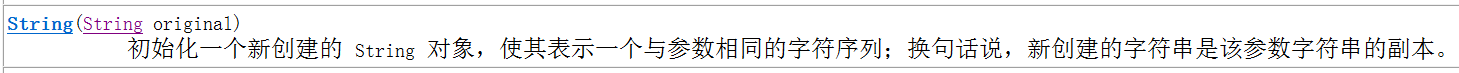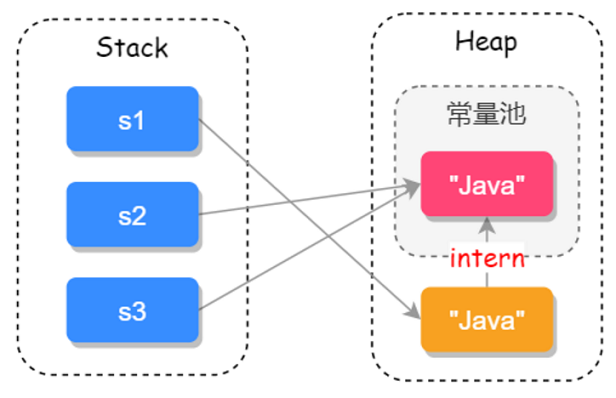2019/04/10 10:10

[toc]

# String的特点？


String s = "abc";   // s引用变量指向了值为"abc"的对象

s = "cba";    // s引用变量又指向了值为"cba"的对象，但是上面值为"abc"的对象的值并未改变




class Demo {
public static void main(String[] args) {
String s1 = "abc";
String s2 = "abc";

Demo d1 = new Demo();
Demo d2 = new Demo();
System.out.println(s1 == s2);     // 1
System.out.println(d1 == d2);
}
}



<font color='red'>String内部实际存储结构为char数组。</font>下面为jdk1.8版本的String源码：


public final class String
implements java.io.Serializable, Comparable<String>, CharSequence {

// 用于存储字符串的值
private final char value[];

// 用于缓存字符串的hashcode
private int hash; // Default to 0

// else code
// ... ...
}




class Demo {
public static void main(String[] args) {
String s1 ="Ja"+"va";
String s2 = "Java";
System.out.println(s1 == s2);
}
}



# String的构造方法？

String是一个类，所以我们除了使用String s1 = "abc"方式创建字符串对象之外，还可以通过String类的构造方法进行创建。通过查看jdk文档我们发现String类 有下面这样的构造函数：class Demo {
public static void main(String[] args) {
String s1 = newString("Java");   // 1
String s2 = s1.intern();         // 2
String s3 = "Java";              // 3
System.out.println(s1 == s2);
System.out.println(s2 == s3);
}
}



1处代码的含义是：在堆内存中使用new的方式创建了一个字符串对象并把地址值赋给了引用变量s1，并且该对象在创建的时候接收了一个字符串对象；而2处代码的含义是：在堆内存中创建一个变量s2，如果调用intern()才会把此字符串保存到字符串常量池中；3处代码的含义是：在字符串常量池中创建了一个值为"Java"的字符串对象，并把该对象的地址值赋给了引用变量s3；所以<font color='red'>3处的代码最终只涉及到一个对象，而1处的代码有可能涉及一个(字符串常量池中有字符串对象"Java")也有可能涉及两个(字符串常量池中没有字符串对象"Java")。</font>这三个引用变量在JVM中存储的位置如下：class Demo {
public static void main(String[] args) {
String s1 = "abc";
String s2 = new String("abc");

System.out.println(s1 == s2);
System.out.println(s1.equals(s2));
}
}




public boolean equals(Object obj) {
return (this == obj);   // 比较的还是对象的地址值
}




public boolean equals(Object anObject) {
// 对象引用相同直接返回 true
if (this == anObject) {
return true;
}

// 判断需要对比的值是否为String类型，如果不是则直接返回false
if (anObject instanceof String) {
String anotherString = (String)anObject;
int n = value.length;
if (n == anotherString.value.length) {
// 把两个字符串都转换为 char 数组对比
char v1[] = value;
char v2[] = anotherString.value;
int i = 0;

// 循环比对两个字符串的每一个字符
while (n-- != 0) {

// 如果其中有一个字符不相等就 true false，否则继续对比
if (v1[i] != v2[i])
return false;
i++;
}
return true;
}
}
return false;
}



String类有几个很常用的构造方法，如下：


// char[]为参数的构造方法
public String(char value[]) {
this.value = Arrays.copyOf(value, value.length);
}

// byte[]为参数的构造方法
public String(byte bytes[]) {
this(bytes, 0, bytes.length);
}

// string为参数的构造方法
public String(String original) {
this.value = original.value;
this.hash = original.hash;
}

// StringBuffer为参数的构造方法
public String(StringBuffer buffer) {
synchronized(buffer) {
this.value = Arrays.copyOf(buffer.getValue(), buffer.length());
}
}

// StringBuilder为参数的构造方法
public String(StringBuilder builder) {
this.value = Arrays.copyOf(builder.getValue(), builder.length());
}



# String的常用方法？

• 用于获取：

1.1 获取字符串中字符的个数(长度)：
int length();
1.2 根据位置获取字符：
char charAt(int index);
1.3 根据字符获取在字符串中的第一次出现的位置：
int indexOf()；    // 注意此方法的有多种重载形式


• 用于转换

1.1 将字符串变成字符串数组(字符串的切割)：
String[]  split(String regex);   // 注意此方法涉及到正则表达式
1.2 将字符串中的字母转成大小写：
String toUpperCase()：大写
String toLowerCase()：小写
1.3 将字符串中的内容进行替换：
String replace();
1.4 将字符串两端的空格去除：
String trim();


• 用于判断

1.1 判断字符串中是否包含指定字符串：
boolean contains(string str);


• 用于比较

1.1 比较两个字符串：
int compareTo(String anotherString)

// 下面为compareTo方法的源码
public int compareTo(String anotherString) {
int len1 = value.length;
int len2 = anotherString.value.length;
int lim = Math.min(len1, len2);
char v1[] = value;
char v2[] = anotherString.value;

int k = 0;

// 对比每一个字符
while (k < lim) {
char c1 = v1[k];
char c2 = v2[k];

// 如果字符不相等就返回差值
if (c1 != c2) {
return c1 - c2;
}
k++;
}
return len1 - len2;
}



0
0 收藏

### 作者的其它热门文章0 评论
0 收藏
0